Resources

# Videos

Learn how to use Squark from start to productive implementation of results.

## Learn what Squark automated machine learning for marketing is all about—creating actionable predictions.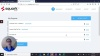## Learn about binary (yes-no), multivariate (which of three or more), and regression (forecast of numerical value) models.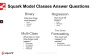## An overview of the AI algorithms used automatically by Squark to find the most predictive model for your data.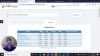## Why you need 1. a training data set (from which Squark learns patterns), and 2. a production data set (containing the records for which Squark will add predictions in the target variable column(s)).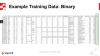## How to load training and production data into Squark.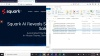## Explanation of selecting columns (features) for modeling and prediction in Squark.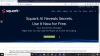## Learn how Squark automatically adds detail to data sets where possible in order to increase their predictive value.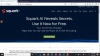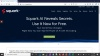## See how Squark results can be understood and put into action.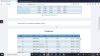## How does Squark determine the rank of each model on the leaderboard for our three different types of analysis?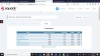## See how Squark reveals which data features (variables) are most predictive so you can inform action plans accordingly.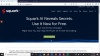## What does the Confusion Matrix show on the result screen when you run a binary or multinomial classification?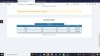## Here is an example of predicting the likelihood of an outcome for each record where there are only two possible answers. Cable subscription data is used. Find the sample churn data sets here for your own practice.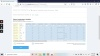## For pricing predictions, Squark automatically uses algorithms the are best for regression, or forecasting numerical values such as price, in this example. Learn how to do it. Download sample pricing datasets here for your own practice.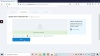## In this example you will learn how to predict the likelihood of offer success when there are three or more possibilities. Download sample datasets for coupon multivariate classification for your own practice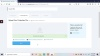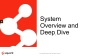### Automated Machine Learning

Learn more about the benefits of automated machine learning as well as the AI power underpinning Squark.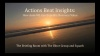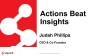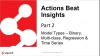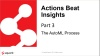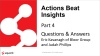### Squark Use Cases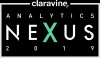## Here is an example of predicting the likelihood of an outcome for each record where there are only two possible answers. Cable subscription data is used. Find the sample churn data sets here for your own practice.## For pricing predictions, Squark automatically uses algorithms the are best for regression, or forecasting numerical values such as price, in this example. Learn how to do it. Download sample pricing datasets here for your own practice.## In this example you will learn how to predict the likelihood of offer success when there are three or more possibilities. Download sample datasets for coupon multivariate classification for your own practice